Related Articles
Unified Modeling Language (UML) | Activity Diagrams
• Difficulty Level : Medium
• Last Updated : 13 Feb, 2018

We use Activity Diagrams to illustrate the flow of control in a system and refer to the steps involved in the execution of a use case. We model sequential and concurrent activities using activity diagrams. So, we basically depict workflows visually using an activity diagram. An activity diagram focuses on condition of flow and the sequence in which it happens. We describe or depict what causes a particular event using an activity diagram.

UML models basically three types of diagrams, namely, structure diagrams, interaction diagrams, and behavior diagrams. An activity diagram is a behavioral diagram i.e. it depicts the behavior of a system.

An activity diagram portrays the control flow from a start point to a finish point showing the various decision paths that exist while the activity is being executed. We can depict both sequential processing and concurrent processing of activities using an activity diagram. They are used in business and process modelling where their primary use is to depict the dynamic aspects of a system.

An activity diagram is very similar to a flowchart. So let us understand if an activity diagrams or a flowcharts are any different :

### Difference between an Activity diagram and a Flowchart –

Flowcharts were typically invented earlier than activity diagrams. Non programmers use Flow charts to model workflows. For example: A manufacturer uses a flow chart to explain and illustrate how a particular product is manufactured. We can call a flowchart a primitive version of an activity diagram. Business processes where decision making is involved is expressed using a flow chart.

So, programmers use activity diagrams (advanced version of a flowchart) to depict workflows. An activity diagram is used by developers to understand the flow of programs on a high level. It also enables them to figure out constraints and conditions that cause particular events. A flow chart converges into being an activity diagram if complex decisions are being made.

Brevity is the soul of wit. We need to convey a lot of information with clarity and make sure it is short. So an activity diagram helps people on both sides i.e. Businessmen and Developers to interact and understand systems.

A question arises:

Do we need to use both the diagram and the textual documentation?
Different individuals have different preferences in which they understand something. For example: To understand a concept, some people might prefer a written tutorial with images while others would prefer a video lecture.
So we generally use both the diagram and the textual documentation to make our system description as clear as possible. We also need to be sensitive to the needs of the audience that we are catering to at times.

Difference between a Use case diagram and an Activity diagram

An activity diagram is used to model the workflow depicting conditions, constraints, sequential and concurrent activities. On the other hand, the purpose of a Use Case is to just depict the functionality i.e. what the system does and not how it is done. So in simple terms, an activity diagram shows ‘How’ while a Use case shows ‘What’ for a particular system.
The levels of abstraction also vary for both of them. An activity diagram can be used to illustrate a business process (high level implementation) to a stand alone algorithm (ground level implementation). However, Use cases have a low level of abstraction. They are used to show a high level of implementation only.Figure – an activity diagram for an emotion based music player

The above figure depicts an activity diagram for an emotion based music player which can also be used to change the wallpaper.

The various components used in the diagram and the standard notations are explained below.

### Activity Diagram Notations –

1. Initial State – The starting state before an activity takes place is depicted using the initial state.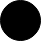Figure – notation for initial state or start state

A process can have only one initial state unless we are depicting nested activities. We use a black filled circle to depict the initial state of a system. For objects, this is the state when they are instantiated. The Initial State from the UML Activity Diagram marks the entry point and the initial Activity State.

For example – Here the initial state is the state of the system before the application is opened.Figure – initial state symbol being used

2. Action or Activity State – An activity represents execution of an action on objects or by objects. We represent an activity using a rectangle with rounded corners. Basically any action or event that takes place is represented using an activity.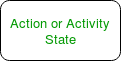Figure – notation for an activity state

For example – Consider the previous example of opening an application opening the application is an activity state in the activity diagram.Figure – activity state symbol being used

3. Action Flow or Control flows – Action flows or Control flows are also referred to as paths and edges. They are used to show the transition from one activity state to another.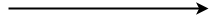Figure – notation for control Flow

An activity state can have multiple incoming and outgoing action flows. We use a line with an arrow head to depict a Control Flow. If there is a constraint to be adhered to while making the transition it is mentioned on the arrow.

Consider the example – Here both the states transit into one final state using action flow symbols i.e. arrows.Figure – using action flows for transitions

4. Decision node and Branching – When we need to make a decision before deciding the flow of control, we use the decision node.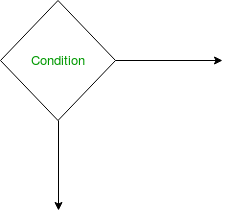Figure – notation for decision node

The outgoing arrows from the decision node can be labelled with conditions or guard expressions.It always includes two or more output arrows.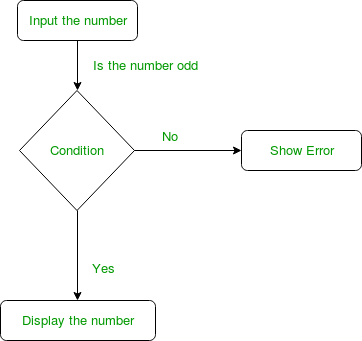Figure – an activity diagram using decision node

5. Guards – A Guard refers to a statement written next to a decision node on an arrow sometimes within square brackets.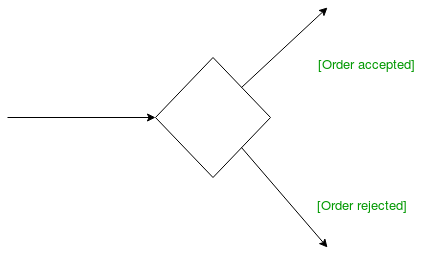Figure – guards being used next to a decision node

The statement must be true for the control to shift along a particular direction. Guards help us know the constraints and conditions which determine the flow of a process.

6. Fork – Fork nodes are used to support concurrent activities.Figure – fork notation

When we use a fork node when both the activities get executed concurrently i.e. no decision is made before splitting the activity into two parts. Both parts need to be executed in case of a fork statement.
We use a rounded solid rectangular bar to represent a Fork notation with incoming arrow from the parent activity state and outgoing arrows towards the newly created activities.
For example: In the example below, the activity of making coffee can be split into two concurrent activities and hence we use the fork notation.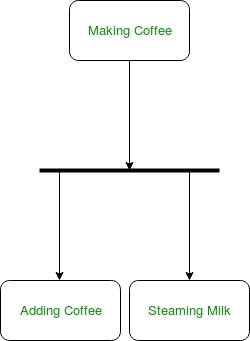Figure – a diagram using fork

7. Join – Join nodes are used to support concurrent activities converging into one. For join notations we have two or more incoming edges and one outgoing edge.Figure – join notation

For example – When both activities i.e. steaming the milk and adding coffee get completed, we converge them into one final activity.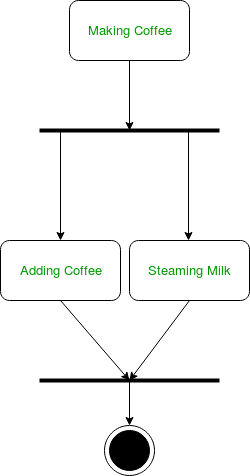Figure – a diagram using join notation

8. Merge or Merge Event – Scenarios arise when activities which are not being executed concurrently have to be merged. We use the merge notation for such scenarios. We can merge two or more activities into one if the control proceeds onto the next activity irrespective of the path chosen.Figure – merge notation

For example – In the diagram below: we can’t have both sides executing concurrently, but they finally merge into one. A number can’t be both odd and even at the same time.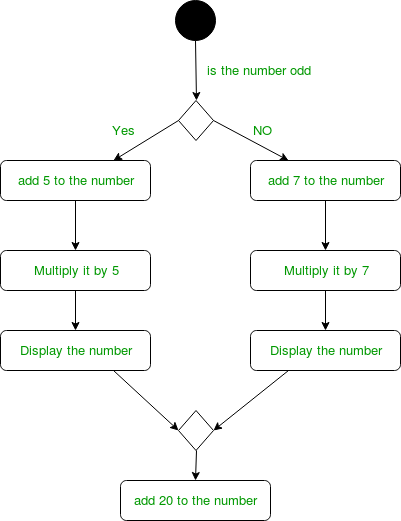Figure – an activity diagram using merge notation

9. Swimlanes – We use swimlanes for grouping related activities in one column. Swimlanes group related activities into one column or one row. Swimlanes can be vertical and horizontal. Swimlanes are used to add modularity to the activity diagram. It is not mandatory to use swimlanes. They usually give more clarity to the activity diagram. It’s similar to creating a function in a program. It’s not mandatory to do so, but, it is a recommended practice.Figure – swimlanes notation
We use a rectangular column to represent a swimlane as shown in the figure above.

For example – Here different set of activities are executed based on if the number is odd or even. These activities are grouped into a swimlane.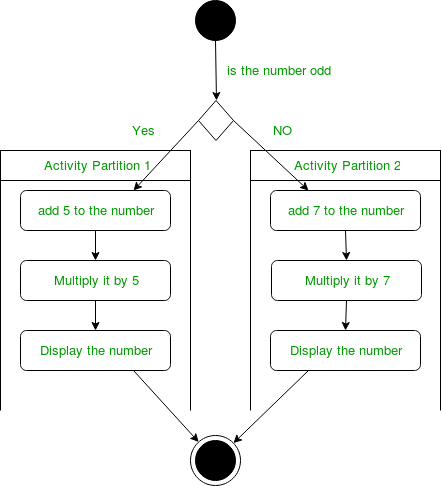Figure – an activity diagram making use of swimlanes

10. Time Event –Figure – time event notation

We can have a scenario where an event takes some time to complete. We use an hourglass to represent a time event.
For example – Let us assume that the processing of an image takes takes a lot of time. Then it can be represented as shown below.Figure – an activity diagram using time event

11. Final State or End State – The state which the system reaches when a particular process or activity ends is known as a Final State or End State. We use a filled circle within a circle notation to represent the final state in a state machine diagram. A system or a process can have multiple final states.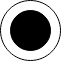Figure – notation for final state

### How to Draw an activity diagram –

1. Identify the initial state and the final states.
2. Identify the intermediate activities needed to reach the final state from he initial state.
3. Identify the conditions or constraints which cause the system to change control flow.
4. Draw the diagram with appropriate notations.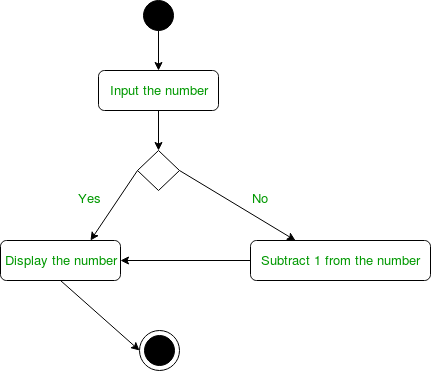Figure – an activity diagram

The above diagram prints the number if it is odd otherwise it subtracts one from the number and displays it.

### Uses of an Activity Diagram –

• Dynamic modelling of the system or a process.
• Illustrate the various steps involved in a UML use case.
• Model software elements like methods,operations and functions.
• We can use Activity diagrams to depict concurrent activities easily.
• Show the constraints, conditions and logic behind algorithms.

This article is contributed by Ankit Jain . If you like GeeksforGeeks and would like to contribute, you can also write an article using contribute.geeksforgeeks.org or mail your article to contribute@geeksforgeeks.org. See your article appearing on the GeeksforGeeks main page and help other Geeks.

Please write comments if you find anything incorrect, or you want to share more information about the topic discussed above.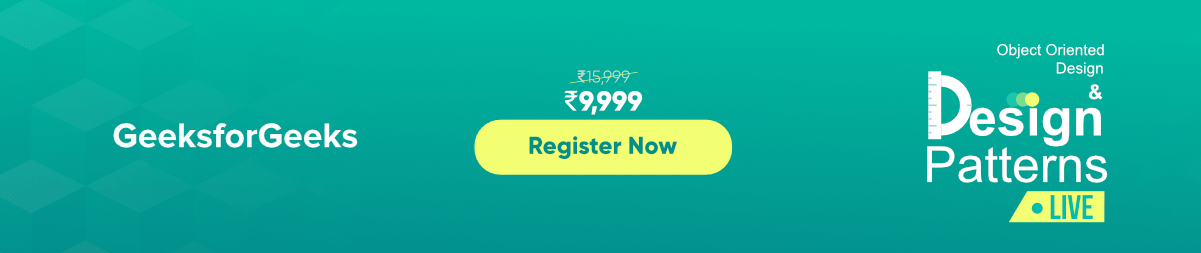My Personal Notes arrow_drop_up